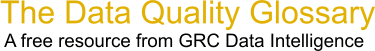Choose Index below for a list of all words and phrases defined in this glossary.

# Probability (P) Value

index | Index

## Probablity (P) Value - definitions

P Value - The probability value (p-value) of a statistical hypothesis test is the probability of getting a value of the test statistic as extreme as or more extreme than that observed by chance alone, if the null hypothesis Ho, is true.

It is the probability of wrongly rejecting the null hypothesis if it is in fact true.

It is equal to the significance level of the test for which we would only just reject the null hypothesis. The p-value is compared with the desired significance level of our test and, if it is smaller, the result is significant. That is, if the null hypothesis were to be rejected at the 5% significance level, this would be reported as "p < 0.05".

Small p-values suggest that the null hypothesis is unlikely to be true. The smaller it is, the more convincing the evidence is that null hypothesis is false. It indicates the strength of evidence for say, rejecting the null hypothesis H0, rather than simply concluding "Reject Ho" or "do not reject Ho".

[Category=Data Quality ]

P-Value - Each statistical test has an associated null hypothesis, the p-value is the probability that your sample could have been drawn from the population(s) being tested (or that a more improbable sample could be drawn) given the assumption that the null hypothesis is true. A p-value of .05, for example, indicates that you would have only a 5% chance of drawing the sample being tested if the null hypothesis was actually true.

Null Hypothesis are typically statements of no difference or effect. A p-value close to zero signals that your null hypothesis is false, and typically that a difference is very likely to exist. Large p-values closer to 1 imply that there is no detectable difference for the sample size used. A p-value of 0.05 is a typical threshhold used in industry to evaluate the null hypothesis. In more critical industries (healthcare, etc.) a more stringent, lower p-value may be applied.

More specifically, the p-value of a statistical significance test represents the probability of obtaining values of the test statistic that are equal to or greater in magnitude than the observed test statistic. To calculate a p-value, collect sample data and calculate the appropriate test statistic for the test you are performing. For example, t-statistic for testing means, Chi-Square or F statistic for testing variances etc. Using the theoretical distribution of the test statistic, find the area under the curve (for continuous variables) in the direction(s) of the alternative hypothesis using a look up table or integral calculus. In the case of discrete variables, simply add up the probabilities of events occurring in the direction(s) of the alternative hypothesis that occur at and beyond the observed test statistic value.

[Category=Data Quality ]

Source: iSixSigma, 10 February 2011 09:51:24, http://www.isixsigma.com/index.php?option=com_glossaryThese advertisers support this free service

p-value - [statistics] A probability resulting from a statistical test of the coefficient associated with each independent variable in a regression model. The null hypothesis for this statistical test states that the coefficient is not significantly different from zero. Small p-values reflect small probabilities. They suggest that the coefficient is significantly different from zero, and consequently, that the associated explanatory variable is helping to model or predict the dependent variable. Variables with coefficients near zero do not help predict or model the dependent variable; they are almost always removed from the regression equation (unless there are strong theoretical reasons to keep them).

[Category=Geospatial ]

Source: esri, 21 July 2012 10:55:29, http://support.esri.com/en/knowledgebase/GISDictionary/term/abbreviationData Quality Glossary.  A free resource from GRC Data Intelligence. For comments, questions or feedback: dqglossary@grcdi.nl